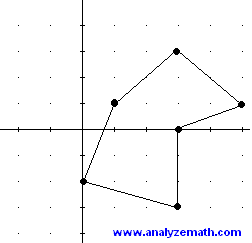# Irregular Polygon Area Calculator

Online calculator to calculate the area of a non-self-intersecting irregular polygon with n vertices given by their Cartesian coordinates. The figure below shows an example of an irregular polygon with 6 vertices.How to use the calculator

Enter the number of points n that form the irregular polygon and the coordinates x and y of the vertices and press "calculate area". The vertices coordinates must be input in order: either clockwise or anticlockwise. The output is the area of the irregular polygon. In case you have a polygon with more than 10 vertices, divide it into smaller polygons find the area of each polygon and add them to obtain the total area.

 n = x1 = , y1 = x2 = , y2 = x3 = , y3 = x4 = , y4 = x5 = , y5 = x6 = , y6 = x7 = , y7 = x8 = , y8 = x9 = , y9 = x10 = , y10 = area =
More Online Geometry Calculators and Solvers.